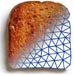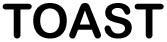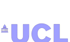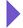Image Reconstruction in Optical Tomography

Home

Introduction
Matlab toolbox

Documentation
Getting Started
Matlab demos
FAQ
Change log
References

# Toast toolbox tutorial: Constructing the Jacobian

The computation of the Jacobian matrix J is required by most gradient-based image reconstruction approaches. The Jacobian contains the derivatives of the measurements with respect to the model parameters. It is a matrix of size M x P, where M is the number of measurement data, and P is the number of model parameters to reconstruct (e.g. the coefficients of a finite-dimensional basis expansion of the absorption and scattering parameters).

J = {∂ yi/∂ xj}

When multiple parameter and data types are present, J has a block structure. For example, if absorption coefficient μa and diffusion coefficient κ are the model parameters, and the data consist of log amplitude lnA and phase φ,

 J = ∂ lnAi/∂ μj ∂ lnAi/∂ κj ∂ φi/∂ μj ∂ φi/∂ κj

Within each block, each line of the matrix describes the effect of perturbing a parameter has on one specific measurement. This is called the Photon Measurement Density Function (PMDF). A PMDF can be displayed as an image.

Toast has a function toastJacobian which returns J for a given set of absorption and scattering parameters and a given set of source and detector definitions. The syntax is

J = toastJacobian(mesh,basis,qvec,mvec,mua,mus,ref,freq);
where mesh is the mesh object, basis is the solution basis mapper, qvec and mvec are the sparse matrices containing the source and detector column vectors, mua, mus, are the absorption and diffusion coefficient vectors in the mesh basis, ref is the refractive index vector in the mesh basis, and freq is the modulation frequency.

The returned matrix is expressed in the solution basis defined by the basis mapper basis. If the results should be expressed in the mesh basis instead, replace the basis object with "0" in the argument list.

To obtain the Jacobian for a steady-state problem, set freq=0. In that case, the bottom half of the returned matrix (containing the phase PMDFs) will be zero. Often in steady-state problems, only the absorption parameter is reconstructed, due to the nonuniqueness of the dual-parameter reconstruction problem. In that case, a simplified real-valued version toastJacobianCW can be used which only returns ∂ lnA/∂ μ (the top left block). The syntax is

J = toastJacobianCW(mesh,basis,qvec,mvec,mua,mus,ref);

This function is more efficient than calling toastJacobian and discarding the unused blocks of the returned matrix.